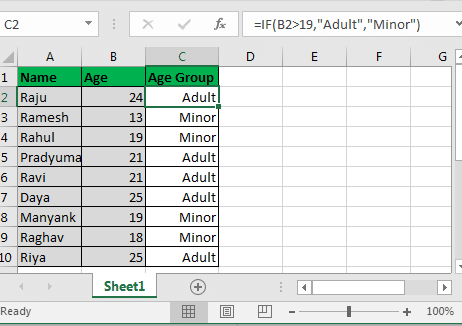# Learning conditional if function in Excel (in very simple language)

The IF function in Excel is used to generate conditional outputs. Command: = IF (condition, value if TRUE, value if FALSE) The IF statement in Excel checks the condition and returns the specified value for each of the answers if it is true…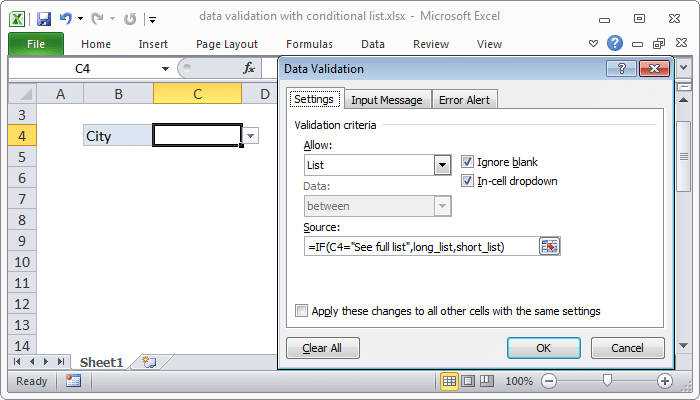# The easiest way to validate data Data validation in Excel

How to prevent space before or after a string? I do not want the user to make a mistake and there is a gap before or after each string. I want to give an error message to…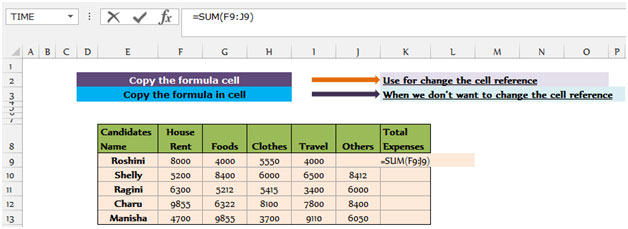# Learn how to copy formulas in Excel in a principled way

Simply put, when we copy the formula from cell to cell, the Draxel cell reference changes. However, when we transfer each formula from cell to cell, the cell reference will be the same. In this article…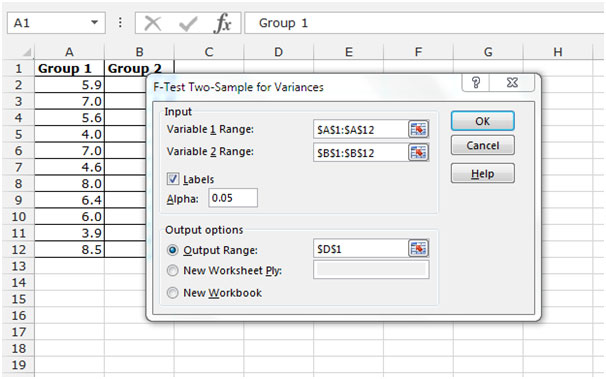# Step-by-step instruction of F-test in Excel in simple language

Array1 , array2, and F-test are not significantly different in the two-sentence distribution used to distinguish between the two sample variance functions.  However, these groups have different levels of test score variability. How to write a test (F: = FTEST…# Learn the easiest way to create random numbers in Excel

Here we will learn how to generate a random number in Microsoft Excel . To generate a random number, we will use the “RAND” and “RANDBETWEEN” functions. The rand function is used to generate a random number greater than or equal to 0 and less than 1,…# Learn the frequency / frequency function in Excel in simple language

FREQUENCY : This function calculates the amount of data in a given interval and then returns a vertical array of numbers with one more element than the binary array. In this section we will learn how…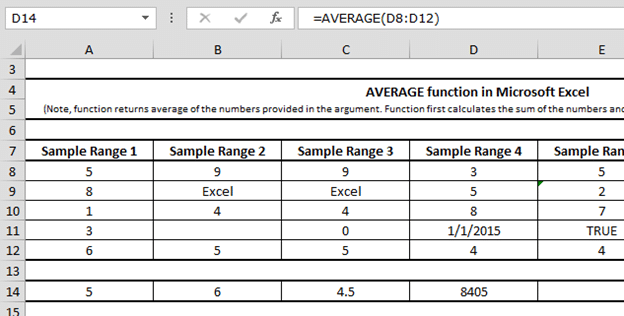# Learning the average function in Excel in a very simple language

The AVERAGE function in Excel is one of the most common statistical functions. This function calculates the mean (arithmetic mean) of the numbers given in the argument. AVERAGE is calculated by adding the number of cells and then dividing by the…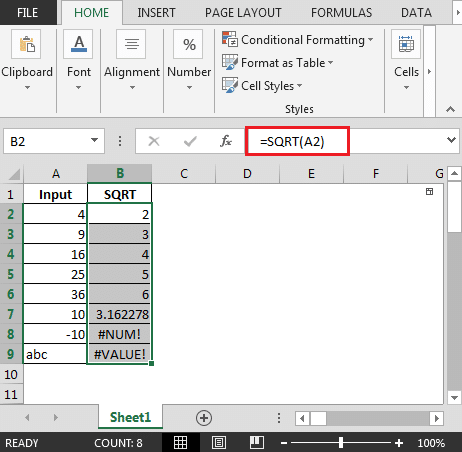# Learn the simplest method of rooting (second root) in Excel

If you want to calculate the square root of a number in Excel, you can use the square formula. In this article, we will learn how to take the square root of a number. You…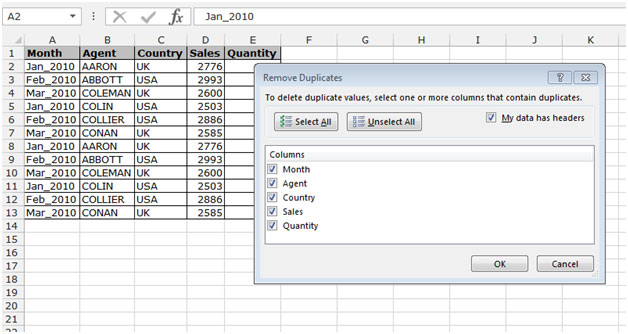# The easiest way to delete duplicate items in Excel

In this article, we will learn how to delete duplicate items in Excel. If our project has a lot of duplicate data, manually deleting it will be a very time consuming and big task. Excel has…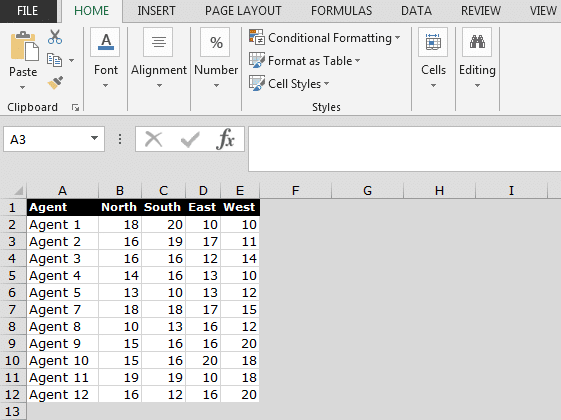# The easiest way to delete empty rows in Excel

This option allows direct access to various cells in Excel. You can enter the cell address in this window and find it quickly. Shortcut key: f5 and Ctrl + G Main path: Home > Find & Select > Go to Special . Let’s…